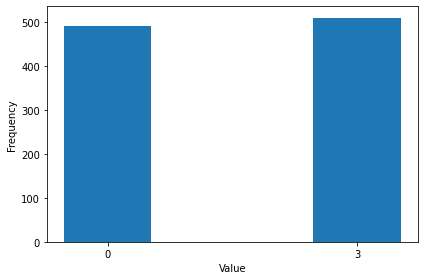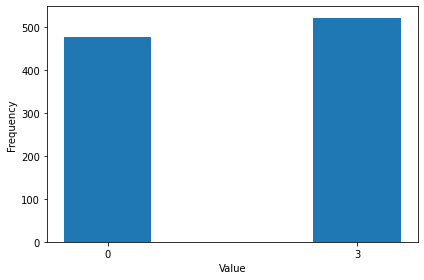# Quantum circuits on Rigetti devices

try:
import cirq
except ImportError:
print("installing cirq...")
!pip install --quiet cirq
print("installed cirq.")

import cirq

print(cirq.__version__)

0.12.0.dev


Running this notebook requires the pyQuil QVM and Compiler. If you are running on Google Colab or a Linux Debian machine, you can run the below cell to install them if necessary. If you are on a non-Linux Debian machine, see these instructions for installation.

! [ -z "$(which qvm)" ] &&\ apt update &&\ apt install jq &&\ export LATEST_FOREST_SDK_VERSION=$(curl -s https://downloads.rigetti.com/qcs-sdk/versions | jq -r '.versions.sdk') &&\
curl -f https://downloads.rigetti.com/qcs-sdk/forest-sdk-$LATEST_FOREST_SDK_VERSION-linux-deb.tar.bz2 -o$PWD/forest-sdk-$LATEST_FOREST_SDK_VERSION-linux-deb.tar.bz2 &&\ tar -xf forest-sdk-$LATEST_FOREST_SDK_VERSION-linux-deb.tar.bz2 &&\
./forest-sdk-$LATEST_FOREST_SDK_VERSION-linux-deb/forest-sdk-$LATEST_FOREST_SDK_VERSION-linux-deb.run &&\
quilc --version &&\
qvm --version


Next, run the pyQuil QVM and Compiler if they are not already running on their default ports.

import subprocess

subprocess.Popen(["qvm", "--quiet", "-S"])
subprocess.Popen(["quilc", "--quiet", "-R"])

<subprocess.Popen at 0x7f40fcff4310>


# Running a Bell state circuit

To demonstrate the basic functionality of the Cirq Rigetti integration, we begin constructing a basic Bell state circuit.

bell_circuit = cirq.Circuit()
qubits = cirq.LineQubit.range(2)
bell_circuit.append(cirq.H(qubits))
bell_circuit.append(cirq.CNOT(qubits, qubits))
bell_circuit.append(cirq.measure(qubits, qubits, key='m'))
print(bell_circuit)

0: ───H───@───M('m')───
│   │
1: ───────X───M────────


Next, we'll import RigettiQCSService and list available quantum processors.

from cirq_rigetti import RigettiQCSService
import json

quantum_processors = RigettiQCSService.list_quantum_processors().quantum_processors
print(json.dumps([quantum_processor.id for quantum_processor in quantum_processors], sort_keys=True, indent=4))

[
"Aspen-9"
]


For now, we'll instantiate the RigettiQCSService as a pyQuil Quantum Virtual Machine based on the topology of one of the available Rigetti quantum processors. At the time of this writing, Aspen-9 is available.

Note, in addition to the quantum processors listed above, you can also instantiate the RigettiQCSService by naming an arbitrary virtual device the pyQuil QVM supports. See the documentation for pyquil get_qc for more information.

from cirq_rigetti import circuit_transformers, circuit_sweep_executors, get_rigetti_qcs_service

service = get_rigetti_qcs_service("Aspen-9", as_qvm=True, noisy=False)
result = service.run(bell_circuit, repetitions=1000)

print(result.histogram(key='m'))

Counter({3: 510, 0: 490})


We'll define a function to visually verify the results of our Bell state.

import matplotlib.pyplot as plt
import numpy as np

def plot_bell_state(result):
labels = list(result.keys())
labels.sort()

values = [result[label] for label in labels]

x = np.arange(len(labels))  # the label locations
width = 0.35  # the width of the bars

fig, ax = plt.subplots()
rects1 = ax.bar(x, values, width, label='Bell state')

# Add some text for labels, title and custom x-axis tick labels, etc.
ax.set_ylabel('Frequency')
ax.set_xticks(x)
ax.set_xticklabels(labels)
ax.set_xlabel('Value')

fig.tight_layout()

plt.show()

plot_bell_state(result.histogram(key='m'))As expected, we see states 0 (ie '00') and 3 (ie '11') as the dominant results.

You may initialize both the RigettiQCSService and RigettiQCSSampler with execution functions from the cirq_rigetti.circuit_sweep_executor module and transformation functions from cirq_rigetti.circuit_transformations.

You may invoke these functions with arguments for controlling your circuit execution at a more fine grained level. For instance, you may want add Pragma statements to set the initial rewiring strategy, invoke active qubit reset prior to execution, or explicitly address physical qubits on the quantum computer.

from pyquil.quilbase import Reset, Pragma

def hook(program, measurement_id_map):
program._instructions.insert(0, Reset())
program._instructions.insert(1, Pragma('INITIAL_REWIRING', freeform_string='GREEDY'))
print(program)
return program, measurement_id_map

# assign qubits explicitly to hardware or virtual machine qubits.
qubit_id_map = {
qubits: 4,
qubits: 5,
}
executor = executor = circuit_sweep_executors.with_quilc_compilation_and_cirq_parameter_resolution
transformer = circuit_transformers.build(qubit_id_map=qubit_id_map, qubits=qubits, post_transformation_hooks=[hook])
service = get_rigetti_qcs_service('Aspen-9', as_qvm=True, executor=executor, transformer=transformer)
result = service.run(bell_circuit, repetitions=1000)

plot_bell_state(result.histogram(key='m'))

RESET
PRAGMA INITIAL_REWIRING "GREEDY"
DECLARE m0 BIT
H 4
CNOT 4 5
MEASURE 4 m0
MEASURE 5 m0# Running a parameterized circuit

Of course, you may be running a parameterized circuit and would like to leverage the Quil compilers's support for parametric compilation. This affords a speedup in execution times as the Cirq Rigetti integration will only compile the circuit once for a single parameter sweep.

We start by initializing the RigettiQCSSampler and specifying a circuit sweep executor that supports parametric compilation. Note, that this class accepts the same executor and transformer types as RigettiQCSService.

from cirq_rigetti import get_rigetti_qcs_sampler

executor = circuit_sweep_executors.with_quilc_parametric_compilation
sampler = get_rigetti_qcs_sampler('Aspen-9', as_qvm=True, executor=executor)


Next, we will initialize a parameterized circuit in Cirq along with a set of parameter values.

import sympy

qubit = cirq.LineQubit.range(1)

circuit = cirq.Circuit(
cirq.H(qubit)**sympy.Symbol('t'),
cirq.measure(qubit, key='m'))
params = cirq.Linspace('t', start=0, stop=4, length=5)

print(circuit)

0: ───H^t───M('m')───


In much the same way that we invoke, RigettiQCSSerivce.run, we invoke RigettiQCSSampler.run_sweep with our parameters here.

import pandas as pd

results = sampler.run_sweep(circuit, params, repetitions=10)
data = {f't={result.params["t"]}': [measurement for measurement in result.measurements['m']] for result in results}

pd.DataFrame(data)

[{ "type": "thumb-down", "id": "missingTheInformationINeed", "label":"Missing the information I need" },{ "type": "thumb-down", "id": "tooComplicatedTooManySteps", "label":"Too complicated / too many steps" },{ "type": "thumb-down", "id": "outOfDate", "label":"Out of date" },{ "type": "thumb-down", "id": "samplesCodeIssue", "label":"Samples / code issue" },{ "type": "thumb-down", "id": "otherDown", "label":"Other" }]
[{ "type": "thumb-up", "id": "easyToUnderstand", "label":"Easy to understand" },{ "type": "thumb-up", "id": "solvedMyProblem", "label":"Solved my problem" },{ "type": "thumb-up", "id": "otherUp", "label":"Other" }]